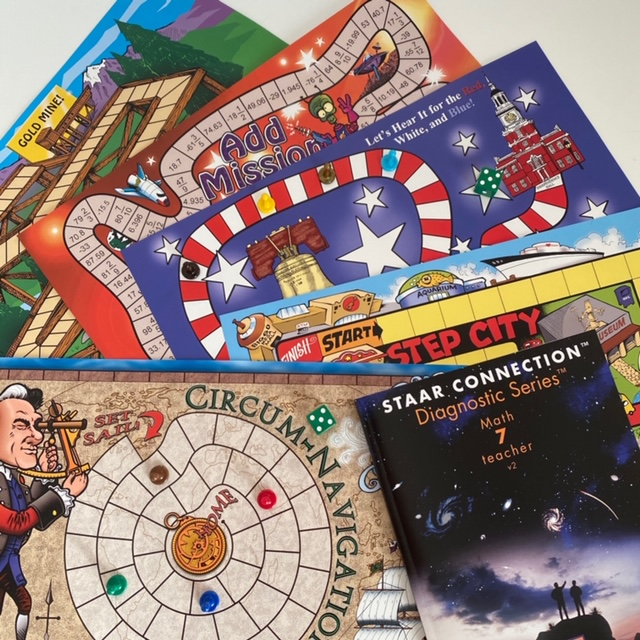#### QUICK START PACK - Grade 7 Math

\$110.00 Qty: (0 in cart)

SKU: QSP7M

Includes the STAAR CONNECTION™ Diagnostic Series™ Grade 7 Math teacher edition; Game Gallery® (9 - 1 of each of the grade 7 TEKS-based math board games) 15% discount

### Details

Quick Start Pack

Math

STAAR CONNECTIONDiagnostic Series

 Item # Description ISBN Qty STDI7MV2T Gr 7 Math v2 - Teacher 978-1-62426-242-5 1

Game Gallery® Gr 7 Math Games

 Item # Game Title and TEKS Qty GG7MAM Add Mission 7(3)(A,B) Number and operations. Add, subtract, multiply, and divide rational numbers fluently. 7(1)(A) Mathematical processes. Apply mathematics to problems arising in everyday life. 1 GG7MA Area 51 7(4)(A) Proportionality. Represent and solve problems involving proportional relationships. 1 GG7MCN Circum-Navigation 7(5)(B) Proportionality. Describe pi as the ratio of the circumference of a circle to its diameter. 1 GG7MLHI Let’s Hear It for the Red, White, and Blue! 7(12)(B) Measurement and data. Use data from a random sample to make inferences about a population. 1 GG7MPP Proportional Prospecting 7(5)(C) Proportionality. Solve mathematical and real-world problems involving similar shape and scale drawings. 1 GG7MRR Ratio Rally 7(4)(B) Proportionality. Calculate unit rates from rates in mathematical and real-world problems. 1 GG7MSTC Sample the Cheese, Please! 7(1)(G) Mathematical processes. Display, explain, and justify mathematical ideas and arguments using precise mathematical language in written communication. 1 GG7MSC Step City 7(1)(B) Mathematical processes. Use a problem-solving model that incorporates analyzing given information, formulating a plan or strategy, determining a solution, justifying the solution, and evaluating the problem-solving process and the reasonableness of the solution. 1 GG7MSS Symbol Slide 7(11)(A) Expressions, equations, and relationships. Model and solve equations. 1